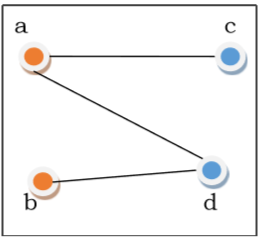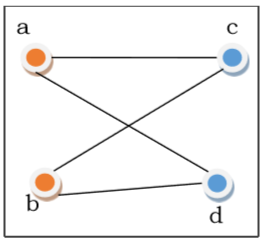# Bipartite Graphs

Bipartite Graph - If the vertex-set of a graph G can be split into two disjoint sets, V1 and V2 , in such a way that each edge in the graph joins a vertex in V1 to a vertex in V2 , and there are no edges in G that connect two vertices in V1 or two vertices in V2 , then the graph G is called a bipartite graph.Complete Bipartite Graph - A complete bipartite graph is a bipartite graph in which each vertex in the first set is joined to every single vertex in the second set. The complete bipartite graph is denoted by Kx,y where the graph G contains x vertices in the first set and y vertices in the second set.